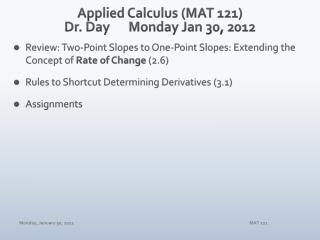# Applied Calculus (MAT 121) Dr. Day Monday Jan 30 , 2012 - PowerPoint PPT PresentationDownload PresentationApplied Calculus (MAT 121) Dr. Day Monday Jan 30 , 2012

Applied Calculus (MAT 121) Dr. Day Monday Jan 30 , 2012Download Presentation## Applied Calculus (MAT 121) Dr. Day Monday Jan 30 , 2012

- - - - - - - - - - - - - - - - - - - - - - - - - - - E N D - - - - - - - - - - - - - - - - - - - - - - - - - - -
##### Presentation Transcript

1. Applied Calculus (MAT 121)Dr. Day Monday Jan30, 2012 • Review: Two-Point Slopes to One-Point Slopes: Extending the Concept of Rate of Change (2.6) • Rules to Shortcut Determining Derivatives (3.1) • Assignments MAT 121

2. Two-Point Slope to One-Point Slope (2.6) • Determining Slope for a Function: Average Rate of Change • Applying the Idea of a Limit to the Slope • What Do We Get? Instantaneous Rate of Change • Practice the Algebra! MAT 121

3. Two-Point Slope to One-Point Slope (2.6) Practice the Algebra! Show how to determine f’(x) for f(x) = x2. • Show the definition of derivative. • Use the given function rule in that derivative definition. • Simplify! Look for opportunity to reduce h/h. • Label and restate your response. MAT 121

4. Shortcut Rules for Determining Derivatives (3.1) • What if the function is a constant function? y = k • What if the function is a power function? y =xn • What if the function includes a coefficient? y = cf(x) • What if the function is the sum or difference of several functions? y = cf(x) + dg(x) Applications of Derivatives MAT 121

5. Shortcut Rules for Derivatives (3.1) • What if the function is a constant function? y = k • The derivative of any constant function is 0: If y = f(x) = k, then y’= f’(x) = 0 MAT 121

6. Shortcut Rules for Derivatives (3.1) • What if the function is a power function? y =xn If y = f(x)= xn, then y’ = f’(x)= nxn-1. MAT 121

7. Shortcut Rules for Derivatives (3.1) • What if the function includes a coefficient? y=cf(x) If y =cf(x), then y’ =cf’(x). MAT 121

8. Shortcut Rules for Derivatives (3.1) • What if the function is the sum or difference of several functions? y=cf(x) + dg(x) If y =cf(x) + dg(x), then y’ = cf’(x) + dg’(x). MAT 121

9. Applications of Derivatives MAT 121

10. Applications of Derivatives MAT 121

11. Assignments WebAssign • Quiz #1 due Sunday night (WA tasks through section 1.4) • 2.6 due tonight • 3.1 due Wednesday night Test #1: Wednesday, Feb 1 • Interactive Practice Test: Online • Test #1: material through 2.6 (Definition of Derivative) • Multiple-Choice and Open-Response • Calculators OK on al portions of this test MAT 121